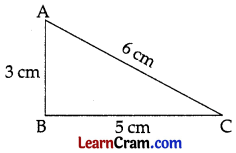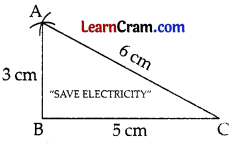# DAV Class 7 Maths Chapter 10 Worksheet 4 Solutions

The DAV Maths Class 7 Solutions and DAV Class 7 Maths Chapter 10 Worksheet 4 Solutions of Construction of Triangles offer comprehensive answers to textbook questions.

## DAV Class 7 Maths Ch 10 WS 4 Solutions

Question 1.
Draw a right triangle with hypotenuse of length 5 cm and one side of length 4 cm.
Steps of Construction: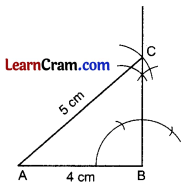1. Draw AB = 4 cm
2. Draw ∠B = 90°
3. Cut AC = 5 cm
4. Join AC
5. ∆ABC is the required right triangle.

Question 2.
Draw a right triangle with hypotenuse of length 4 cm and one side of length 2.5 cm. Measure the other two angles.
Steps of Construction: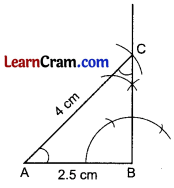1. Draw AB = 2.5 cm
2. Draw ∠B = 90°
3. Cut AC = 4 cm
4. ∆ABC is the required right triangle.
5. ∠A = 46° and ∠B = 44°Question 3.
Construct a right triangle ABC in which AB = 5.8 cm, BC = 4.5 cm and ∠C = 90°.
Steps of Construction: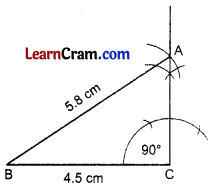1. Draw BC = 4.5 cm
2. Draw ∠C = 90°
3. Taking B as centre, draw an arc of 5.8 cm, which cuts the right angle line at A.
4. Join AC.
5. ∆ABC is the required right triangle.

Question 4.
Construct a right triangle, having hypotenuse of length 5.4 cm and one of the acute angles of measure 30°.
Steps of Construction: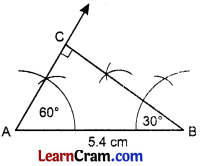1. Draw AB = 4.5 cm
2. Draw ∠B = 30°
3. Draw angle A = 60°
[∵ ∠A = 90° – ∠B = 90° – 30° = 60°]
4. The two angle lines meet at C.
5. ∆ABC is the required right triangle.

Question 5.
Construct a right triangle whose two sides containing the right angle measure 9 cm and 12 cm.
Steps of Construction:1. Draw AB = 12 cm
2. Draw ∠B = 90°
3. Cut BC = 9 cm
4. Join AC
5. ∆ABC is the required right triangle.

### DAV Class 7 Maths Chapter 10 Value Based Questions

Question 1.
Construct a triangular poster of measurement 3 cm, 5 cm, 6 cm. Write the slogan “SAVE ELECTRICITY” on the poster.
(i) What should be done to save electricity?